# PhenoEvoR-intro-vignette

library(PhenoEvoR)

# Analyzing Pheno-Evo data

You’ve run an exeriment using the Pheno-Evo model; you conducted a parameter sweep with BehaviorSpace and exported it as a table. Now what do you do with that table? Here’s a introduction to how PhenoEvoR can help you understand what happened in your model.

To get the full advantage of this tutorial, please download the sample data from our website: PhenoEvo_Example_Experiment.csv. This is a relatively small dataset, so using it should be pretty quick. It was generated from an experiment in which we tested 3 values each of toxin.conc and env.noise, and ran for only 1, timesteps.

## Import and basic processing

Because Pheno-Evo datasets are usually pretty large, we recommend using the fread() function from the data.table package, which deals efficiently with big files. Of course, change the path name below to match the location of PhenoEvo_Example_Experiment.csv on your computer.

PhenoEvoData<-data.table::fread('~/Desktop/PhenoEvo_tutorial/PhenoEvo_Example_Experiment.csv', skip=6, header=T, sep=',', check.names=T)

skip=6 is necessary because NetLogo tables come with a 6-row header.

Inspect the column names of the dataframe:

colnames(PhenoEvoData)
#>   "X.run.number."                "toxin.conc"
#>   "env.noise"                    "X.step."
#>   "count.turtles"                "mean..toxin..of.patches"
#>   "X.response.error..of.turtles" "X.barcode..of.turtles"
#>  "X.generation..of.turtles"     "X.x.y.dr..of.turtles"

They’re not very R-friendly, so we’ll rename them. Caution: if you change the experiment design, make sure to customize this column-renaming step!

colnames(PhenoEvoData)<-c('run.number','toxin.conc','env.noise',
'step','count.turtles','mean.toxin',
'barcode','generation','xydr')

Now, make a list of all the run numbers in your dataset.

run.numbers<-unique(PhenoEvoData\$run.number)

## Creating a final-timepoint dataset

And most of the analyses we’ll do require only the final timepoints of the experiment. So we can make a small and convenient dataframe containing just those timepoints.

PE.ends<-extract.endpoint(PhenoEvoData, run.numbers)

We can make this dataframe even more useful by calculating population-level statistics. If you carry out an Pheno-Evo experiment as in our tutorial, there will be some variables for which you’ll end up storing data on each individual cell at each timepoint. You can calculate the population means of those variables using the summarize.endpoint() function. But first, you need to make a list of the traits you want to summarize.

pop.level.traits<-c('degrade.rate','switch.rate','response.error','generation')

You might notice that PE.ends also has a “barcode” identifier for each cell lineage, as well, but it makes no sense to take the mean of that. The summarize.endpoint() function returns PE.ends with new columns attached. We could make it a new dataframe, but instead we’ll just replace PE.ends.

PE.ends<-summarize.endpoint(PE.ends, pop.level.traits)

Finally, we’ll calculate four metrics of functional diversity for Degrade Rate at the final timepoint of each model run in our experiment. We’ll do that with a function that employs the dbFD function from the FD package (read more in that package’s documentation). There may be some metrics that are not calculable, depending on the structure of your data; you’ll receive an error message, but it won’t harm the calculation of the other metrics. It’s important to note that the trait name has to be given in quotation marks. This function generates a new dataframe, with a column for each of the diversity metrics.

dr.fundiv<-calc.fun.div(PE.ends, 'degrade.rate')
#> FRic: Only one continuous trait or dimension in 'x'. FRic was measured as the range, NOT as the convex hull volume.
#> FDiv: Cannot not be computed when 'x' contains one single continuous trait or dimension.
summary(dr.fundiv)
#>    run.number      nbsp            FRic             FEve
#>  Min.   :1    Min.   :23.00   Min.   :0.8273   Min.   :0.3674
#>  1st Qu.:3    1st Qu.:35.00   1st Qu.:1.2754   1st Qu.:0.4214
#>  Median :5    Median :51.00   Median :1.9647   Median :0.4617
#>  Mean   :5    Mean   :51.11   Mean   :1.9877   Mean   :0.4826
#>  3rd Qu.:7    3rd Qu.:72.00   3rd Qu.:2.8265   3rd Qu.:0.5488
#>  Max.   :9    Max.   :78.00   Max.   :3.4124   Max.   :0.6269
#>       FDis
#>  Min.   :0.1458
#>  1st Qu.:0.2702
#>  Median :0.3355
#>  Mean   :0.3486
#>  3rd Qu.:0.4956
#>  Max.   :0.5533

To make downstream analyses easier, let’s merge the functional diversity results with our PE.Ends dataframe, and remove the functional diversity dataframe from our environment.

PE.ends<-merge(PE.ends, dr.fundiv, by='run.number')
rm(dr.fundiv)

# Plotting results

That’s all the prep you need before you can begin visualizing your results!

## Final-timepoint population means

Because we ran a parameter sweep and would like to know how the values of two parameters, toxin.conc and env.noise, affect the final population that evolves, let’s start with some heatmaps comparing population-level means of some important variables across parameter values.

For all the heatmap functions, the arguments are: * ends.df = the dataframe with endpoint data, after you’ve calculated the mean values as above * xvar = the variable you want on the x-axis (no quotation marks!) * yvar = the variable you want on the y-axis (no quotation marks!) and for survival heatmap and phenotype histogram, you also have the option of * nums = T/F whether to label each block with its run number so you can better identify your data. The default is False.

Let’s start by examining how long each experimental population survived. In some cases, populations may go extinct before the end of the experiment, and that is worth knowing. (Of course, this isn’t technically a mean value, but rather a population-wide number.)

survival.heatmap(PE.ends, xvar=toxin.conc, yvar=env.noise, nums=T)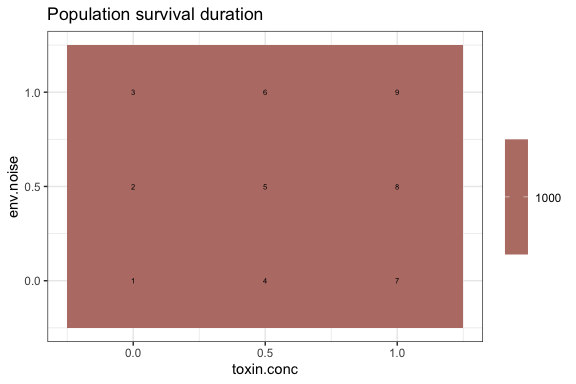However, in this case, this is pretty boring, because all populations survived until the end of the experiment.

Another measure of success is how many generations of cell births elapse during the course of an experiment:

generation.heatmap(PE.ends, xvar=toxin.conc, yvar=env.noise)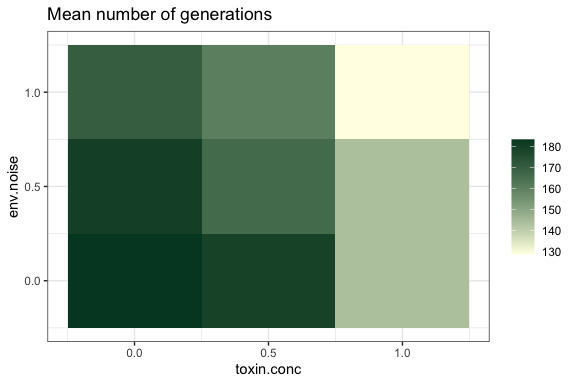This way we can see that even though all the populations survived for 1,000 timesteps, some of them achieved an average of >180 generations per cell lineage in that time, whereas some others only achived 130 generations. As one might expect, populations with more toxin grew less overall. The relationship with environmental noise is perhaps a bit less intuitive, though.

We can also look at population-average degrade rates. Remember that this is a phenotypic trait– in this experiment, it reflects a behavioral response to the environment, not evolutionary adaptation.

degrade.rate.heatmap(PE.ends, xvar=toxin.conc, yvar=env.noise)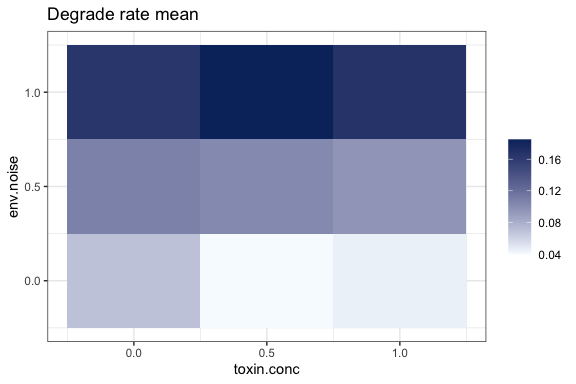Populations tend to react with higher degrade rates not in response to higher toxin, but in response to higher environmental noise. This is one of the not-so-intuitive outcomes of this experiment.

Here is the rate at which cells switch phenotype. This is an evolved, genotypic trait.

switch.rate.heatmap(PE.ends, xvar=toxin.conc, yvar=env.noise)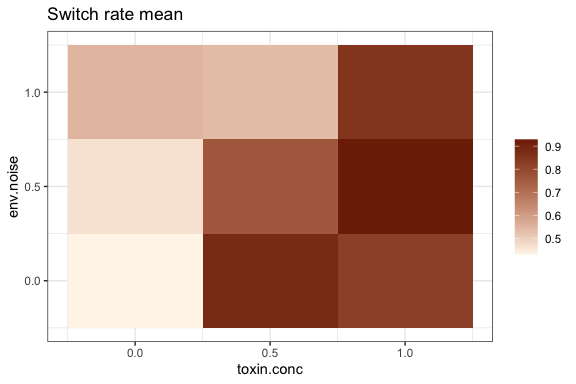In general, populations seem to evolve quicker phenotype-switching in response to higher toxin concentrations.

Here is the cells’ response error– that is, how much flexibility they allow in their response to environmental conditions. Low response error means that cells spend a lot of energy to make sure that they match their phenotype precisely to the toxin level they detect.

response.error.heatmap(PE.ends, xvar=toxin.conc, yvar=env.noise)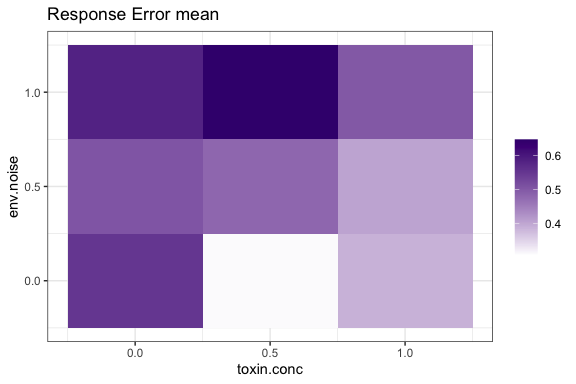Intuitively, it makes sense that when the environment is noisier (less predictable), it’s beneficial to evolve a high response error– that way you’re not wasting energy trying to understand what’s going on in an environment that is fundamentally impossible to understand.

There is also a generic heatmap function that can be used to plot other metrics from your endpoints table, for instance, the functional diversity metrics we calculated for degrade rate. For this, you need to specify an additional argument, * gradientvar = the name of the feature you want the gradient to show

phenoevo.heatmap(PE.ends, xvar=toxin.conc, yvar=env.noise, gradientvar=FDis)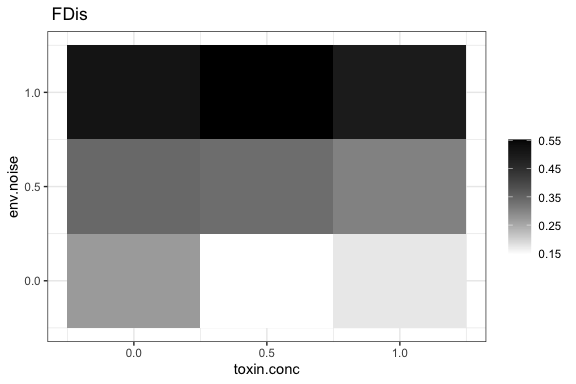Here, we see that there is greater Functional Dispersion of degrade rates in environments with higher environmental noise.

If you want to add your own color code, you can simply write over the current one by adding it on!

phenoevo.heatmap(PE.ends, xvar=toxin.conc, yvar=env.noise, gradientvar=FDis) + ggplot2::scale_fill_gradient(low='#ffffe5', high='#004529', name='')
#> Scale for 'fill' is already present. Adding another scale for 'fill', which
#> will replace the existing scale.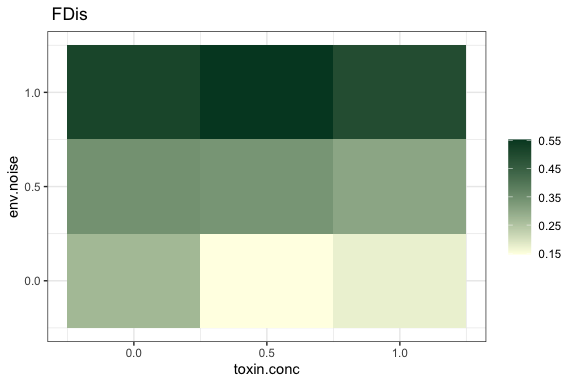See ggplot2 documentation for adding other features. You can pretty much add anything you want to, to the plots produced here.

It’s very important to remember that these are population averages; our plots can’t say anything about the diversity present in the population. Closer analysis is necessary to figure out what’s really going on. In addition, we seem to have recognized some trends, but there is also noise in those trends, likely due to the stochasticity that’s inherent to evolution. It would be worthwhile doing replicates of these experiments, and/or testing at intermediate values across our parameter gradients. If you do recognize a trend, you can easily use the data from the PE.ends dataframe for simple linear modeling, or any other form of statistical analysis you prefer. Finally, it should be acknowledged that these snapshots capture only a single timepoint, yet these populations are constantly in flux. In the long run it would be useful to develop some methods to examine the dynamic steady state of the population.

## Final-timepoint population distributions

The whole point of our project is that just calculating the average value of a diverse population can’t tell you the whole story! So, of course, you’ll want to plot a histogram of degrade rate at the final timepoint for each experiment:

phenotype.histogram(PE.ends, xvar=toxin.conc, yvar=env.noise, nums=F)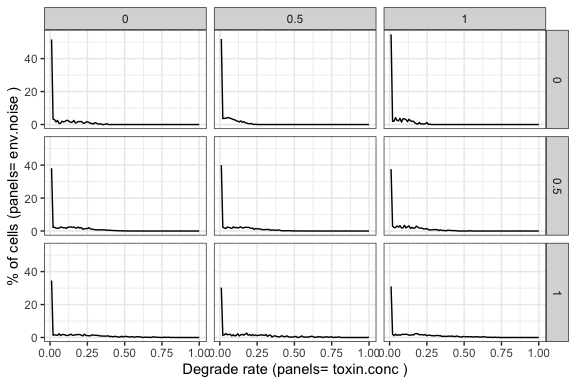Here, you can observe something important that we didn’t get from the summary statistics: ALL populations have a substantial peak of sensitive (low-degrade-rate) cells, even if the population mean value of degrade.rate varies systematically with certain parameters.

You can visualize and analyze spatial heterogeneity as well.Our analyses focus on phenotypic degrade rate. To take a quick look at what the distribution of degrade.rate looks like in the population at the final timepoint of a single experiment, (for instance, here we choose Run Number 4,) you can use the following function to essentially re-plot the population with all cells at their coordinates:

degraderate.snapshot(PE.ends, run.num=4)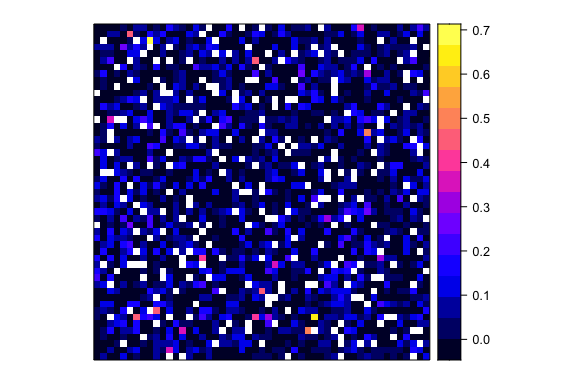Note that the color gradient is entirely different from the one used in the Pheno-Evo model. This function uses the spatial analysis package sp, and its function spplot.

You may want to do quantitative calculations relating to spatial autocorrelation. We’ve included a function for variogram calculation, using the gstat package. This returns a dataframe:

vario.4<-degraderate.variogram(PE.ends, run.num=4)
summary(vario.4)
#>        np              dist            gamma             dir.hor     dir.ver
#>  Min.   : 10299   Min.   : 1.176   Min.   :0.005466   Min.   :0   Min.   :0
#>  1st Qu.: 67524   1st Qu.: 6.268   1st Qu.:0.005514   1st Qu.:0   1st Qu.:0
#>  Median :110192   Median :11.743   Median :0.005550   Median :0   Median :0
#>  Mean   : 96430   Mean   :11.804   Mean   :0.005613   Mean   :0   Mean   :0
#>  3rd Qu.:132954   3rd Qu.:17.323   3rd Qu.:0.005673   3rd Qu.:0   3rd Qu.:0
#>  Max.   :151901   Max.   :22.759   Max.   :0.006068   Max.   :0   Max.   :0
#>     id
#>  var1:15
#>
#>
#>
#>
#> 

And the variogram can be plotted with a simple command:

plot(vario.4)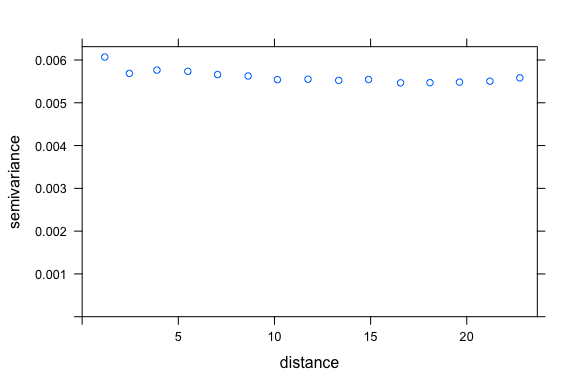For the moment, that’s the extent of our capacity for spatial analysis. However, you can build on this by comparing variograms across different timepoints or parameters. If you want to get more deeply into spatial analysis, we urge you to look at the code for degraderate.snapshot() and degraderate.variogram() as a guide for how to coerce Pheno-Evo data into the right format for use with the sp and Gstat packages; you are welcome to build from there.

## Plotting timecourse data

It can be very informative to see how a population has been behaving over time, though impractical to look at all the populations at once, if you’ve done an experiment that involves many of them. Now that you’ve looked at summary statistics, there may be a few populations you’re particularly interested in. (We’ll use pop 4 just for an example.) The following code allows you to look at how particular properties of your population have changed over time, for a single experiment. All plotting functions require as arguments: * NLdata = your full dataset * runnum = the run number of the experiment of interest. If you’ve already separated your data into individual dataframes by run number, simply enter that dataframe and run number as input - but make sure the dataframe has a column entitled “run.number” or else the subset function will fail.

Here is a standard line plot showing the abundance of cells over time (in red) and the mean concentration of toxin over time (in blue) If the timecourse is longer than 1,000 ticks, only the final 500 ticks are plotted.

cell.abundance.timecourse(PhenoEvoData, runnum=4)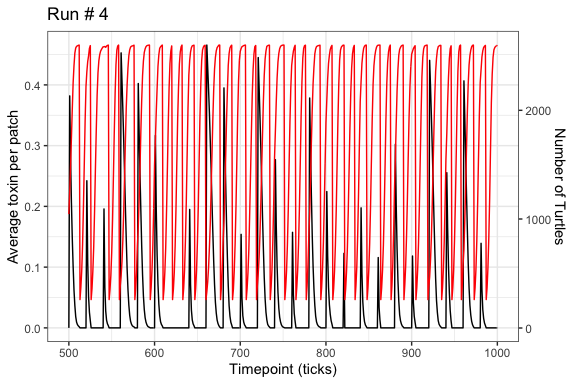Below are heatmaps, showing the distribution of population characteristics at each timepoint. The x-axis is time; the y-axis is the range of potential values of the characteristic (0-1), and the color indicates the proportion of the population that carries that value at that timepoint. These do involve a lot of data, so they can take a little while to render. We’ve tried these plots for timecourses of up to 10,000 timepoints. (More might get unwieldy.) If there are more than 1,000 timepoints, only every 10th timepoint will be plotted.

degrade.rate.timecourse(PhenoEvoData, runnum=4)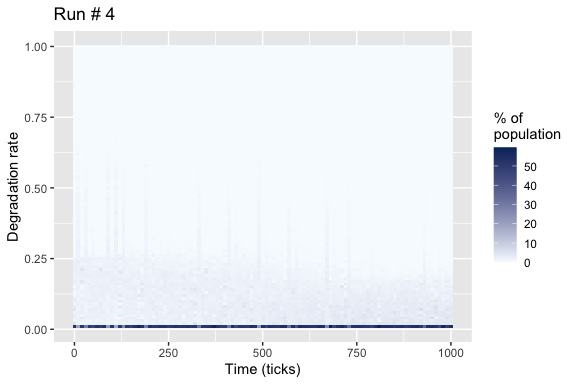You’ll notice some of these plots can be pretty boring… But at least it roughly matches what we saw in the single-timepoint histograms above: most of the population is concentrated at a very low degrade rate.

We can also look at genotype features as they evolve. For instance, response error over time:

response.error.timecourse(PhenoEvoData, runnum=4)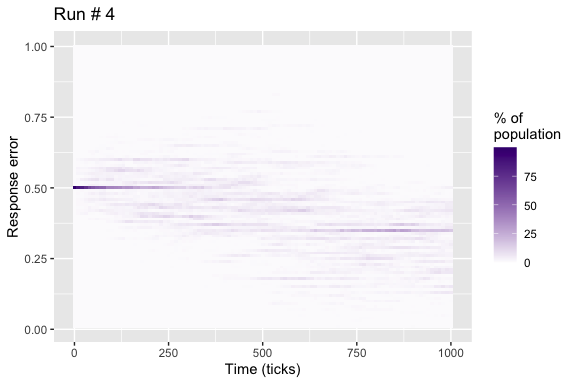And switch rate.

switch.rate.timecourse(PhenoEvoData, runnum=4)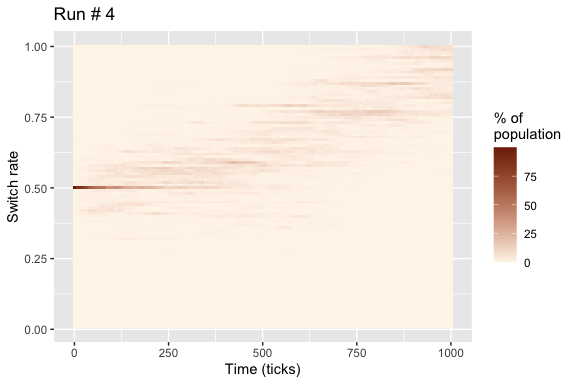With a mutation rate of 0.1, the population drifts pretty quickly.

Finally, we can examine the abundance of each barcode lineage over time. When a model is initialized, each cell receives a unique barcode, and it passess that barcode on to all its progeny. So we can look at the distribution of barcodes in the population across time and get an idea of which lineages were most successful. Note that if the data has more than 1,000 timepoints, only every 10th is plotted. And not every barcode gets its own color. We use a 12-part colorscheme and repeat it.

barcode.timecourse(PhenoEvoData, runnum=2)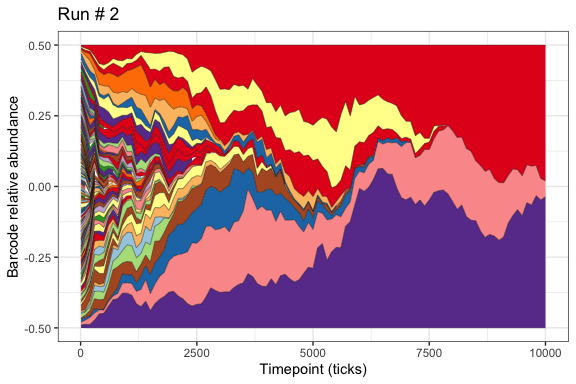Although we start out with 20 distinct lineages, by the end of the experiment only 2 remain. Because there are never any new barcodes introduced in this experiment, but barcode lineages can go extinct due to the toxin-induced death, it makes sense that the population inevitably ends up being dominated by just one. We also know that, because mutations can happen in any cell, the barcode lineage disribution tells us nothing about the genotypes or phenotypes present in the population. So this analysis is of limited value. However, one feature that is sometimes worth noticing is just how quickly barcode distributions change in general in the population, and how this varies with the dilution rate you use.